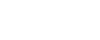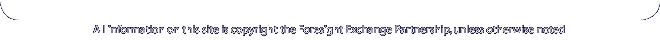Content-Type: text/html; charset=ISO-8859-1 Foresight Exchange Conjuncts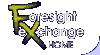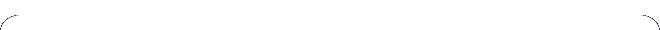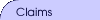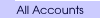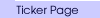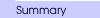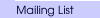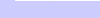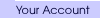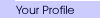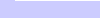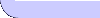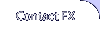Conjuncts are not currently implemented. This draft is a discussion document. Please direct discussion to fx-discuss@ideosphere.com

[Also see "Conjunctive coupons primer" from the Hypermail archive]

• used Perl notation (&& for AND, || for OR) to prevent confusion when coding this, (and to leave a symbol for P(A|B) ).
• P(A|B) means the probability of A occurring given that B has already occurred.
• ~B means NOT B (i.e., the NO coupons pay off)

## WHY Conjuncts?

### Higher Expected Return

If the odds of claim A paying off are 10%, but it ultimately it does, you make 10 times your money. But if instead you buy the AND claim A with another claim B which also has 10% odds, you make 100 times your money.

That's a pretty good reason to be interested in conjuncts.

### A Implies B (A --> B)

This is a bet that B must follow A. You may not know how likely "real" artificial intelligence is (Call it TngT for Turing Test), but may think it is a good bet that a computer will be the world chess champion (Ches) before TngT is true. In other words TngT cannot be true unless Ches is true. Thus would like to:

```         SELL Ches && ~TngT
```

In all cases but where Ches is FALSE and TngT is TRUE, this pays off, assuming both claims are resolved.

```Payoff Matrix:

| Ches ~Ches |
-------+------------+
TngT |  1     1   |
|            |
~TngT |  0     1   |
-------+------------+
```

A market of "imply" conjuncts can be used to determine the consensus on the interrelationship of future developments, so that a critical path chart could be developed. Ideally one could bet on combinations of implies, to bet on the configuration of the whole chart at once (with a huge ROI if you get it right).

Here's a more complicated example: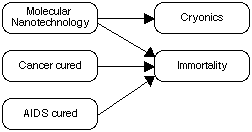### Relative Probability

... trading "p of X if B" for "X if A&&B" is, for a risk-neutral trader, betting that the conditional probability of A given B is greater than p."

(P. 8, Information Prizes: Patronizing Basic Research, Finding Consensus", Robin Hanson, version presented at Western Economics Association meeting, June 1993)

## Theory of Conjuncts

### Bayes' Theorem

"The probability of two dependent events occurring is the probability of the first, multiplied by the conditional probability that the second occurs given the first has already occurred." C.D.J. Waters

```P(A && B) = P(A) * P(B|A)
= P(B) * P(A|B)
P(A|B) = ( P(B|A) * P(A) ) / P(B)
```